Students can download 4th Maths Term 1 Chapter 1 Geometry Ex 1.2 Questions and Answers, Notes, Samacheer Kalvi 4th Maths Guide Pdf helps you to revise the complete Tamilnadu State Board New Syllabus, helps students complete homework assignments and to score high marks in board exams.

## Tamilnadu Samacheer Kalvi 4th Maths Solutions Term 1 Chapter 1 Geometry Ex 1.2

A. Fill in the blanks.

(i) All closed four sided figures are called _______.

(ii) A _______ has four equal sides and equal diagonals.
square

(iii) The opposite sides of a _______ are equal.
rectangle.(iv) A _______ has no sides.
circle

(v) Diagonals are equal in _______ and _______.
square, rhombus

B. Write the name of the sides and digonals.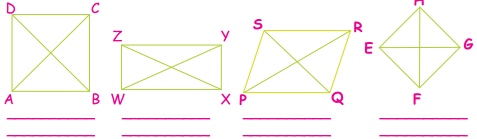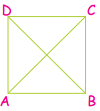Sides = AB, BC, CD, AD
Diagonals = AC, BD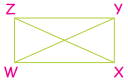Sides = WX, XY, YZ, ZW
Diagonals = WY, XZ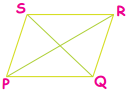Sides = PQ, QR, RS, SP
Diagonals = PR, QS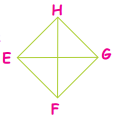Sides = EF, FG, GH, EH
Diagonals = EG, FH